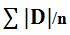# The Mean Absolute Deviation

## Introduction

• Ram, Arjun, Ravi, Ashok are three friends of the same class and they are awaiting their exam results. All of them got 85% marks which were shocking as their response to the different papers was different, few had written English very well and few had written it bad. So how did this happen?
• Let us have a look at their scorecard of individual subjects which is out of 100:
Subject/Student English Hindi Maths Science Total percentage
Ram 70 96 85 89 85
Arjun 99 45 99 97 85
Ravi 82 88 80 90 85
Ashok 60 82 99 99 85
• It is well known that the total percentage is the average percentage of each subject. Though the total percentage of all the four is the same, the scoring pattern of each of them is very different from each other.
• Hence, Average or Mean gives us detail about the overall picture only and it skips the individual contribution of all the elements.
• In other words, Average gives us information about the size or value of elements of the dataset (total percentage) and not about the spread of the values in elements i.e. how much or how less is the contribution of an element (percentage in each subject).
• The measure of dispersion helps us to overcome the drawback of the Mean observed above, it helps in understanding the contribution of each element in a dataset.
• Dispersion is a measure to find out the extent to which values on element differ from the Mean of dataset i.e. in the above example measure of dispersion will give us an idea that how much score did Ram got in each subject (how much or how less than 85).
• There are various ways to measure the dispersion of a dataset which we will study in upcoming sections.

## Mean Absolute Deviation

• Mean Absolute Deviation is a method used to measure the dispersion of a dataset. It is clear from the name itself that it implies the deviation from the mean i.e. how much an element of the dataset has deviated from its mean.
• However, instead of telling the distance of each element from the Mean, it tells the average distance of each element from its mean. It is the average of the distance of each element from the mean. Absolute implies considering only the value and not the sign.

#### Example 1:

• Let us take a dataset: 2,5,6,8,10,12,14,15 and calculate its Mean Absolute Deviation
• Solution: Mean of the data set = (2+5+6+8+10+12+14+15)/8 = 72/8 =9
• Let us find the absolute difference of each element from the mean, here absolute implies considering only the value and not the sign.
Element X Deviation from Mean |D|
2 7
5 4
6 3
8 1
10 1
12 3
14 5
15 6
• Let us take the Mean of the Deviation = (7+4+3+1+1+3+5+6)/8 = 30/8 = 3.75
• The Above value is known as Mean Absolute Deviation.
By looking at the above example, we can conclude the following formula for Mean Absolute Deviation:
• Mean Absolute Deviation =• where n is the total number of elements of a data set
• |D| = Difference between each element of the dataset and Mean of the dataset.